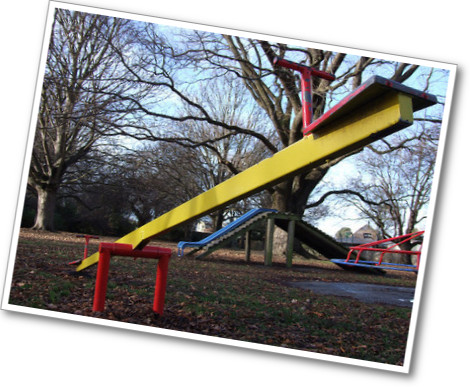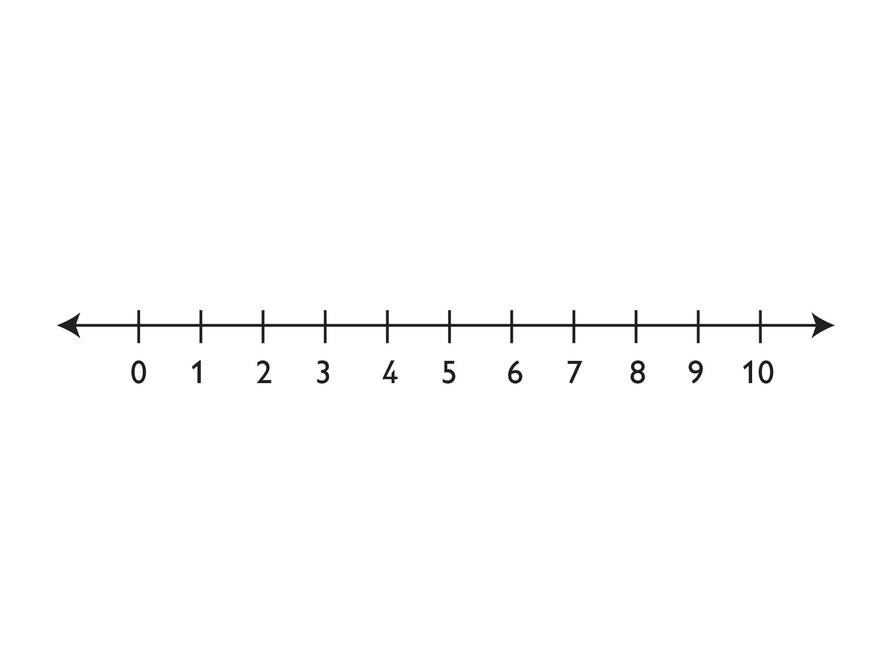# Gallery Problems Exercise# Keep It Balanced

Work with a partner.

1. Take turns finding the card that belongs in the equation to make it a true equation.

3. When you both agree, write the appropriate card into the equation.

HANDOUT: Card Sort

# Equation Sort

1. Sort the equations into three groups:

Equations with only one solution

Equations with many solutions

Equations with no solutions

2. Explain how you know where the equation should be placed.

3. Take one of the equations and change it so that it has a different number of solutions.

Equations:

2x + 5 = 16

6(x + 1) = 6x + 6

3(x + 4) = 3x + 4

2x + 3x = 2x + 12

x + 5 = 5 + x

5 + 4x = 15

2x + 8 = 2x + 3

2x + 7 = 2x + x

3x + 8 = 20

3x – 6 = 6 – 3x

x + 3 = 6 + x

x + 2 + x + 1 = 2x + 3

# On the Number Line

Look at the portion of the number line shown. Use it to help you do problems 1–3. Either copy the number line to your Notebook, or draw your own number line to show your answers.

1. Find a value for j that makes $\frac{4}{j}<1$ true.
• Mark this value of on a number line.
• Is your value the only one possible? If so, say why. If not, describe the set of possible values.

2.  Find a value for k that makes $\frac{4}{k}>1$ true.

• Mark this value of on a number line.
• Is your value the only one possible? If so, say why. If not, describe the set of possible values

3. Find a value for that makes $\frac{4}{m}=1$ true.

• Mark this value of on a number line.
• Is your value the only one possible? If so, say why. If not, describe the set of possible values.# How Many Colors?

A child sees a box of blocks in a store. The child notices these things:

• There are four different shapes.
• There are two different sizes.
• There are several different colors.
• There are 40 blocks in the box.
• There is exactly one block of each “shape-size-color” combination.
1. Write an equation to find the number of colors in the box.

# Value of s

• Show an efficient way to find the value of s + 249 = 47,921.
• Explain why your method works to find the value of s.

# Marbles in a Cup

Chen has some marbles that are all identical.

He puts 12 marbles in a cup. The marbles and the cup together have a mass of 147 grams.

When he puts 15 marbles in the cup, he finds that the marbles and the cup together have a mass of 165 grams.

Let n = the number of marbles in the cup. Let m = the mass of a marble in grams. Let c = the mass of the cup in grams.

1. Write a formula for w, the mass in grams of the cup and the marbles together, using just the variables but no numbers.
2. What is the mass of the cup in grams? Show how you got your answer.

# When Is It True?

1. When is this equation true? Always? Sometimes? Or never?
a + 0 = 1 • a​​​​​​​
2. Show or explain why.